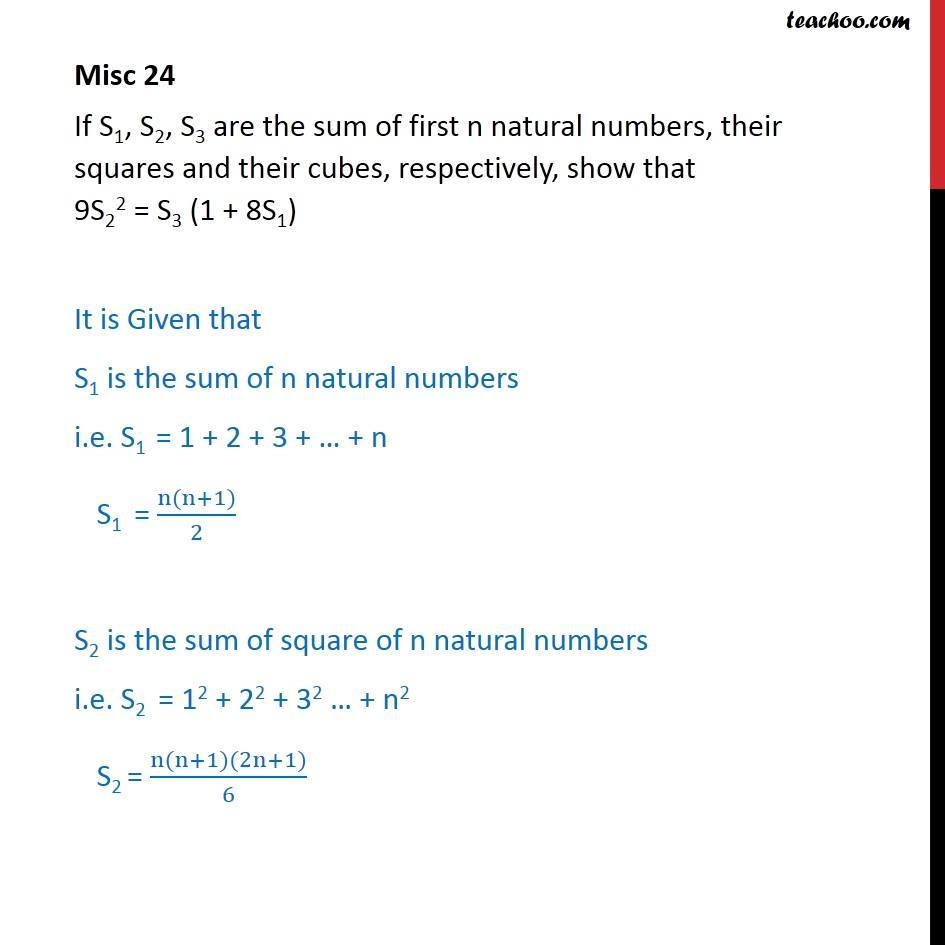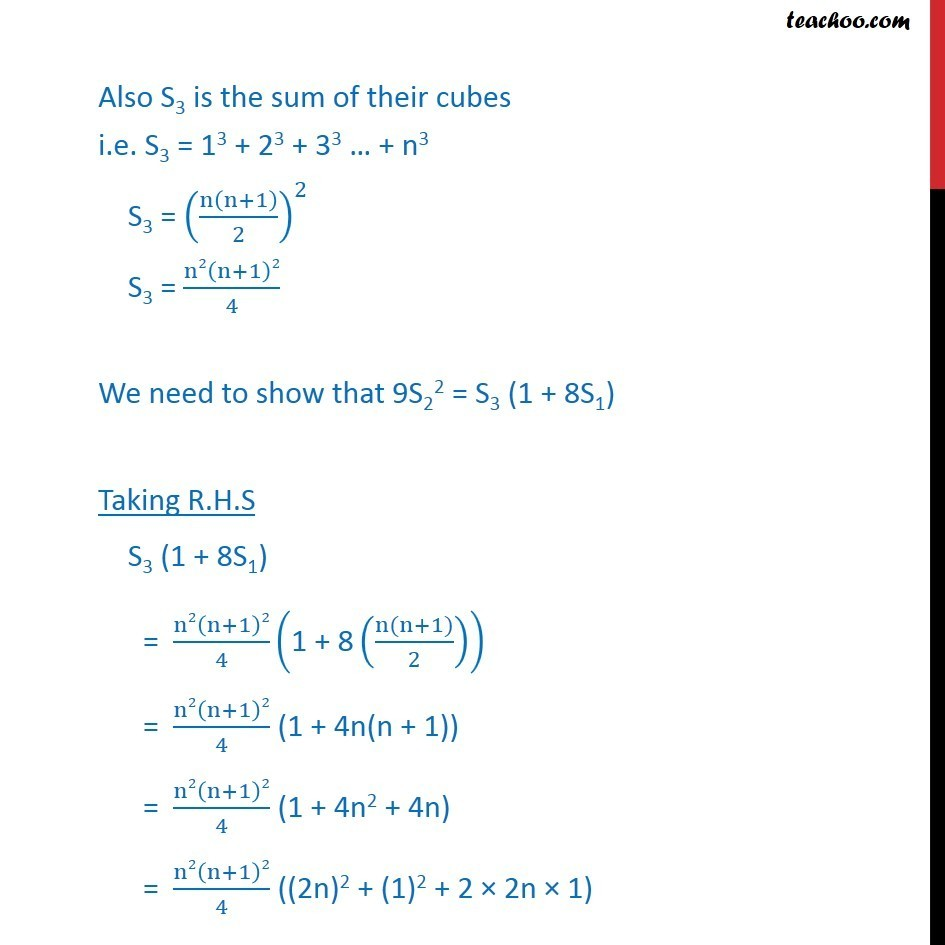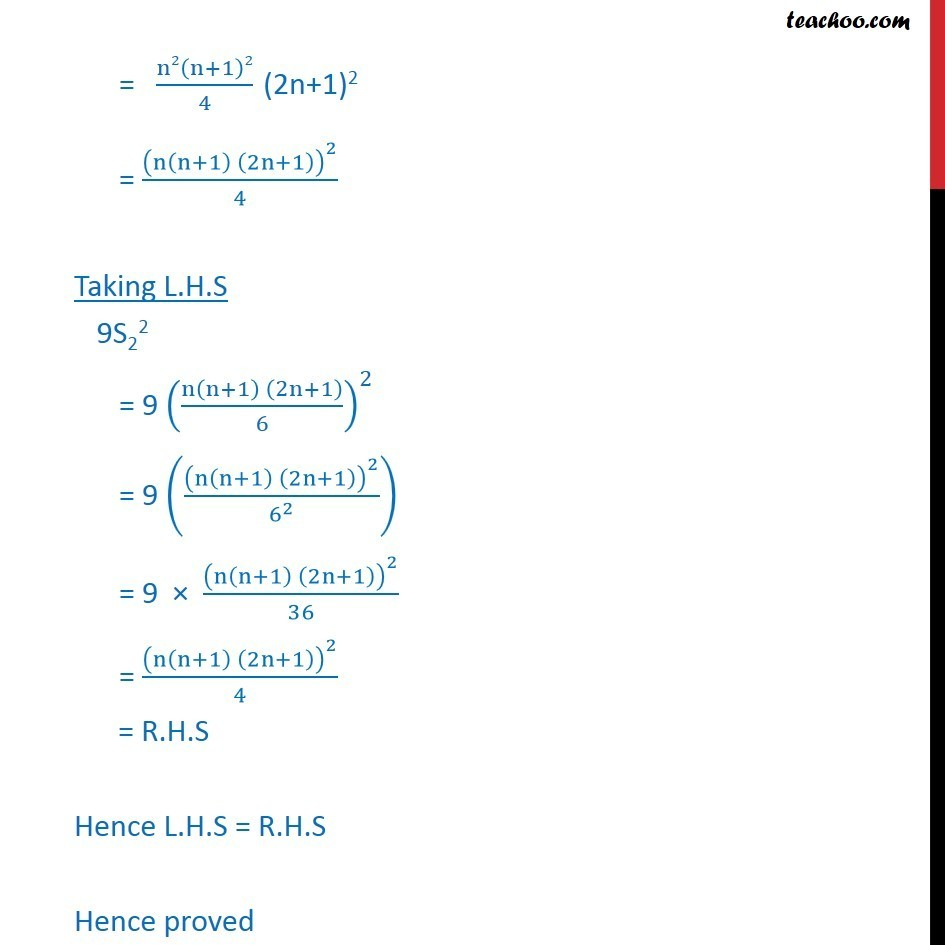Miscellaneous

Chapter 8 Class 11 Sequences and Series
Serial order wiseLearn in your speed, with individual attention - Teachoo Maths 1-on-1 Class

### Transcript

Question 12 If S1, S2, S3 are the sum of first n natural numbers, their squares and their cubes, respectively, show that 9S22 = S3 (1 + 8S1) It is Given that S1 is the sum of n natural numbers i.e. S1 = 1 + 2 + 3 + + n S1 = (n(n+1))/2 S2 is the sum of square of n natural numbers i.e. S2 = 12 + 22 + 32 + n2 S2 = (n(n+1)(2n+1))/6 Also S3 is the sum of their cubes i.e. S3 = 13 + 23 + 33 + n3 S3 = (n(n+1)/2)^2 S3 = n2(n+1)2/4 We need to show that 9S22 = S3 (1 + 8S1) Taking R.H.S S3 (1 + 8S1) = n2(n+1)2/4 ("1 + 8" ((n(n+1))/2)) = n2(n+1)2/4 (1 + 4n(n + 1)) = n2(n+1)2/4 (1 + 4n2 + 4n) = n2(n+1)2/4 ((2n)2 + (1)2 + 2 2n 1) = n2(n+1)2/4 (2n+1)2 = (n(n+1) (2n+1))^2/4 Taking L.H.S 9S22 = 9 ((n(n+1) (2n+1))/6)^2 = 9 ((n(n+1) (2n+1))^2/6^2 ) = 9 (n(n+1) (2n+1))^2/36 = (n(n+1) (2n+1))^2/4 = R.H.S Hence L.H.S = R.H.S Hence proved